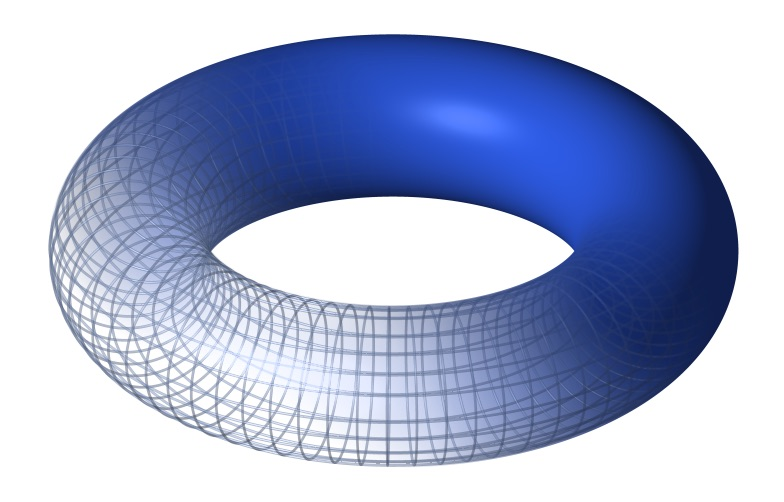# toroidToroid
(click to enlarge)

In mathematics, a toroid is a surface of revolution with a hole in the middle, like a doughnut. The axis of revolution passes through the hole and so does not intersect the surface. For example, when a rectangle is rotated around an axis parallel to one of its edges, then a hollow rectangle-section ring is produced. If the revolved figure is a circle, then the object is called a torus. The term "toroid" Is also used to describe a toroidal polyhedron. In this context a toroid need not be circular and may have any number of holes. Wikipedia, Toroid